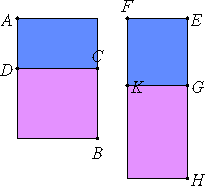# Proposition 26

A medial area does not exceed a medial area by a rational area.

If possible, let the medial area AB exceed the medial area AC by the rational area DB.

Set out a rational straight line EF. Apply to EF the rectangular parallelogram FH equal to AB producing EH as breadth. Subtract the rectangle FG equal to AC. Then the remainder BD equals the remainder KH.

But DB is rational, therefore KH is also rational.

Since each of the rectangles AB and AC is medial, and AB equals FH while AC equals FG, therefore each of the rectangles FH and FG is also medial.X.22

They are applied to the rational straight line EF, therefore each of the straight lines HE and EG is rational and incommensurable in length with EF.

X.20

Since DB is rational and equals KH, therefore KH is rational. And it is applied to the rational straight line EF, therefore GH is rational and commensurable in length with EF.

X.13

But EG is also rational, and is incommensurable in length with EF, therefore EG is incommensurable in length with GH.

X.11

And EG is to GH as the square on EG is to the rectangle EG by GH, therefore the square on EG is incommensurable with the rectangle EG by GH.

But the squares on EG and GH are commensurable with the square on EG, for both are rational, and twice the rectangle EG by GH is commensurable with the rectangle EG by GH, for it is double it, therefore the sum of the squares on EG and GH is incommensurable with twice the rectangle EG by GH.

Therefore the sum of the squares on EG and GH plus twice the rectangle EG by GH, that is, the square on EH is incommensurable with the sum of the squares on EG and GH.

X.Def.I.4

But the squares on EG and GH are rational, therefore the square on EH is irrational.

Therefore EH is irrational. But it is also rational, which is impossible.

Therefore, a medial area does not exceed a medial area by a rational area.

Q.E.D.

## Guide

#### A modern version of the proof

It’s hard to follow this proof. An equivalent proof in modern terms is easier to comprehend. Let √a and √b be the areas of the larger and smaller rectangles, where both are irrational numbers but a and b are both rational numbers.

Suppose that √a – √b is a rational number. Then its square a – 2√(ab) + b is also rational. Then √(ab) is rational, as is √(a/b). Then √a – √b = √b(√(a/b) – 1) is the product of an irrational number and a rational number making it irrational, a contradiction. (For the modern proof, we assume a is not equal to b, so that √(a/b) – 1 does not equal 0, and then we can conclude that the product is irrational.)

This is an interesting proof in that in order to show the difference is irrational, we assumed it was rational and used that to prove it was irrational.

The same modern proof works for sums, of course.

#### Use of this proposition

This proposition is used in several others in Book X starting with X.42.